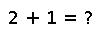# lgamma

``````..  lgamma(x)

Compute the logarithm of the absolute value of :func:`gamma` for
:obj:`Real` ``x``, while for :obj:`Complex` ``x`` it computes the
logarithm of ``gamma(x)``.``````

## Examples

Checking you are not a robot: